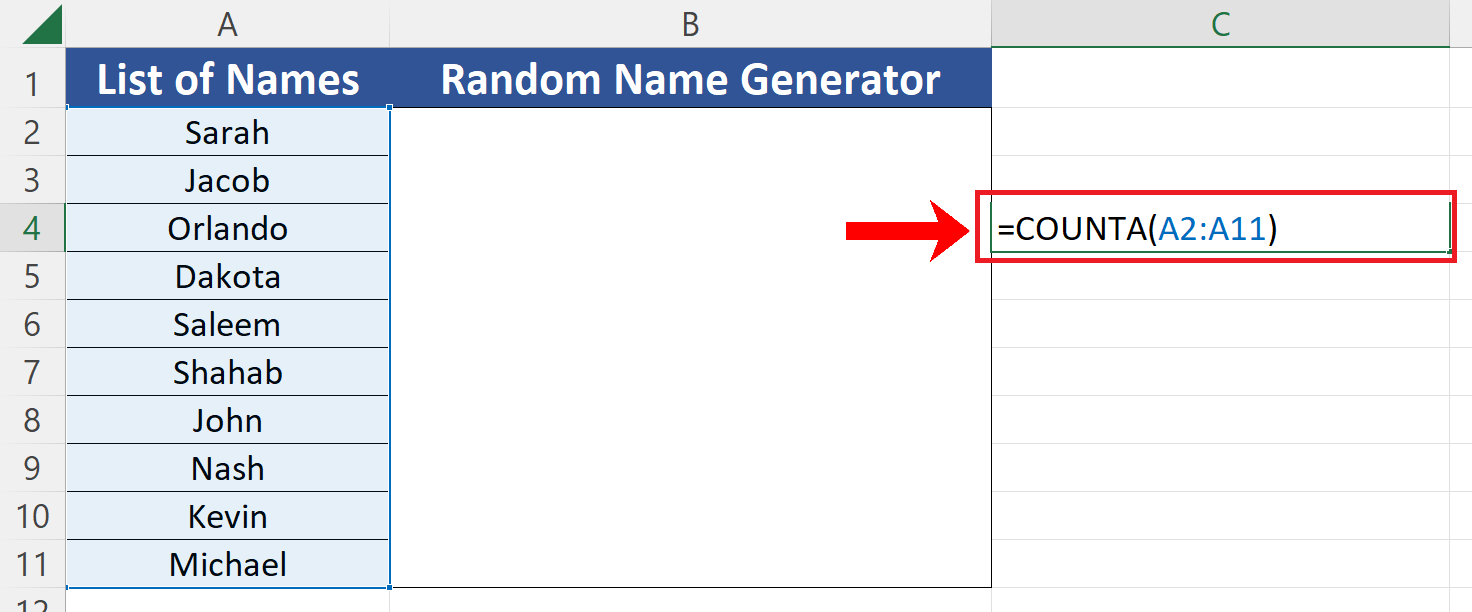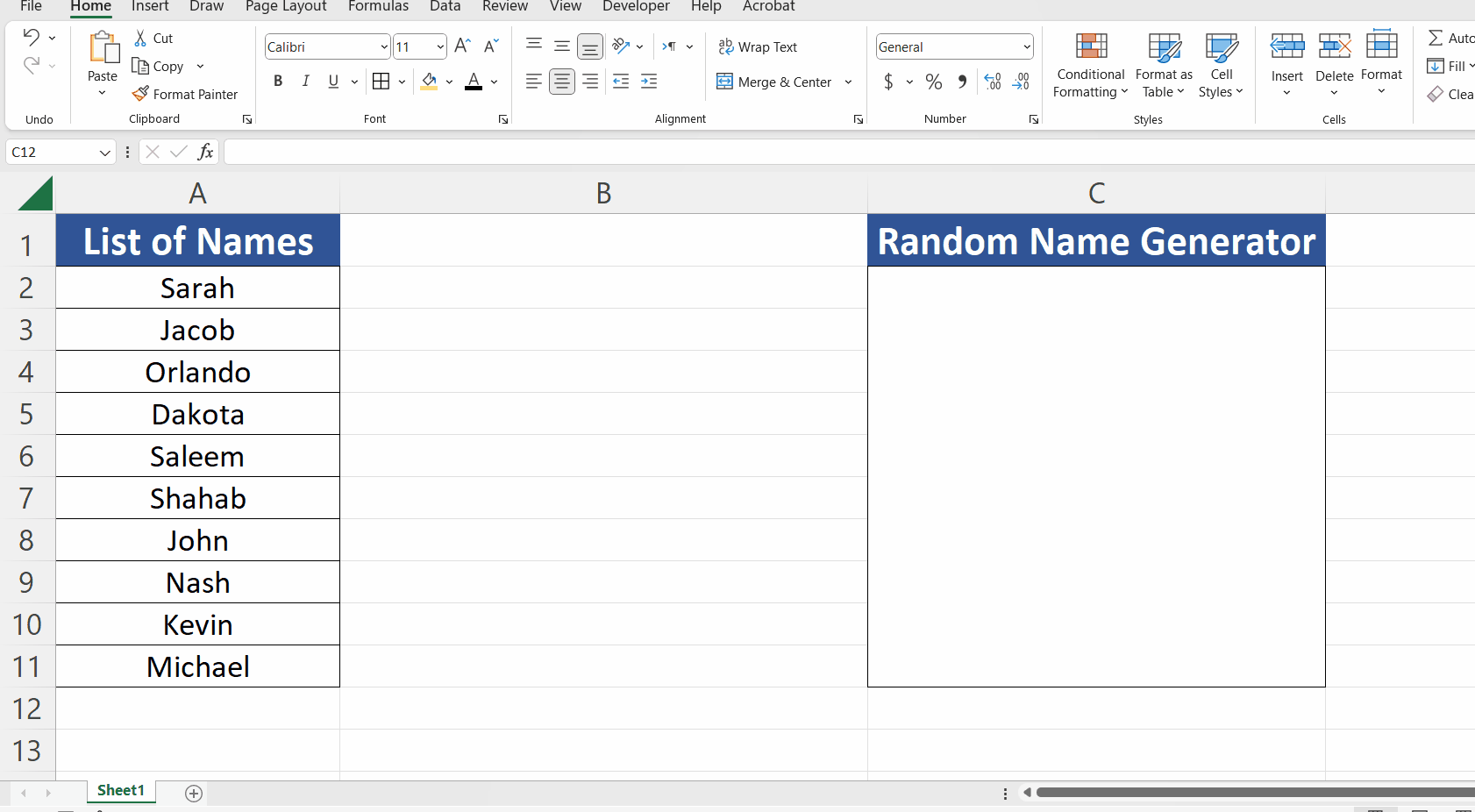# How to generate a random name in Excel

In today’s tutorial, we’ll learn how to generate a random name from the list of names. Let’s look at this dataset. We have the names in the list and we’ll use a combination of CHOOSE and RANDBETWEEN function to create a random name generator, by following these steps.

CHOOSE function picks an item from all the items passed as arguments, using index number which specifies the index of the value to be picked. The syntax of the function is as follows;

=CHOOSE(index_num, value1, [value2], …)

RANDBETWEEN generates an integer number between the bottom and top values which are passed as arguments to this function. The syntax is as follows;

=RANDBETWEEN(bottom,top)We’ll use RANDBETWEEN will generate a random number between 1 and 10 and based on this random value CHOOSE will pick a random name from the list.Excel has built-in functions to generate random numbers which can used for various further reasons. However, there are no direct functions available to generate random text data or very specifically random names from a list of names. Sometimes, we need a random name generator to create names for use in a game or simulation. This can be useful if you want to create a diverse and realistic simulation of a population.

### Step 1 – Find the total number of values in the list– We have a very short list of names just for the sake of explaining this document, otherwise, it could be a very long list of employees. So, to know the total number of entries in the list, use the following formula;
=COUNTA(A2:A11)
For your case replace A2:A11 with the actual range of your dataset. In our case the count is 10.

### Step 2 – Use CHOOSE and RANDBETWEEN to create the formula– Now we’ll use the CHOOSE and RANDBETWEEN functions to create the following formula;
=CHOOSE(RANDBETWEEN(1,10), A2,A3,A4,A5,A6,A7,A8,A9,A10,A11)
– The most important thing to note here is that we’ll have to select all the cells in the range separately, clicking one by one on each of them while holding down the CTRL key as shown below, otherwise this will not work.
– After writing the formula press enter and this will implement the formula. Now to generate the new name press F9 KEY. This will generate a new name from the list each time.